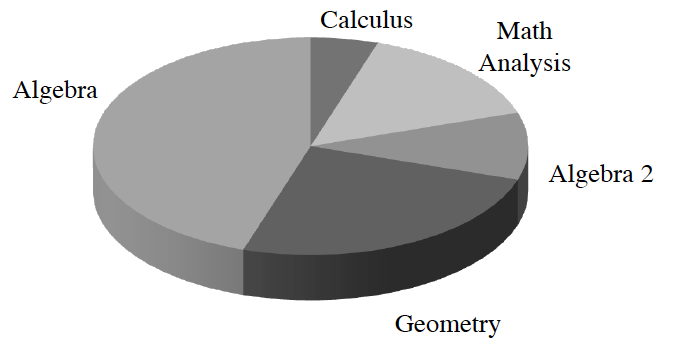### Home > ACC7 > Chapter 8 Unit 9 > Lesson CC3: 8.2.1 > Problem8-51

8-51.

Enrollment in math courses at Kennedy High School in Bloomington, Minnesota is shown in the circle graph at right. (If you are unfamiliar with circle graphs, refer to the glossary located in the eBook for assistance.) If there are $1000$ students enrolled in math courses, approximately how many students are enrolled in Algebra? In Geometry? In Calculus?What approximate percentage of the circle graph is taken up by Algebra, Geometry, and Calculus?

Algebra: $450$
Geometry: $250$
Calculus: $50$